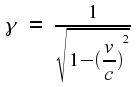# Special Relativity

## Introduction

Albert Einstein published his famous paper on relativity in 1905, so it has had plenty of time to penetrate the public consciousness. Nevertheless, it is not well understood.

Sometimes you will even hear statements to the effect that only half a dozen people in the world understand relativity. This is complete rubbish. The theory is routinely taught to university students, and presumably understood by them. It is true that the topic known as General Relativity is somewhat challenging, and is studied only by advanced students, but that is a further development that was not covered by Einstein's early work on relativity. In these notes I hope to convince you that Special Relativity, the subject of the 1905 paper, is not at all difficult to understand. Some mathematical calculations are required, it is true, but I hope to convince you that it is not necessary to go beyond high school algebra.

## What is relativity?

Relativity, in the sense that the word is used here, refers to the simple idea that absolute position and speed (with respect to the universe, for example) are not necessarily meaningful concepts. What matters is the relative motion between two or more objects and/or observers.

Suppose that a car travelling at 80 km/h is rear-ended by another car travelling at 90 km/h. At what speed did the collision happen? The figures just given are speeds relative to the road, and it might well happen that the drivers' subsequent reactions were affected by the road-relative speed; but, for the purpose of predicting the immediate damage sustained by the vehicles, the important figure is 90-80=10 km/h. That was the relative speed of the two cars. The speed relative to the road is, at this stage, unimportant. It is also unnecessary to take into account the effect of the earth's rotational speed, the speed of the earth relative to the sun, the speed of the sun with respect to the galaxy, and so on.

Of course, it is possible that the collision could distract the driver to the extent that the first car runs into a wall. That would indeed be an 80 km/h collision, because that is the car's speed relative to the wall. Again, however, the relevant speed is a relative speed.

This form of relativity has been well understood since at least the time of Isaac Newton. In fact, a lot of it was understood at the time of Galileo Galilei. In that respect, Einstein added nothing new. Einstein's great contribution was connected with the speed of light.

## Light and the ether

One of my enduring childhood memories is of seeing someone chopping wood in the distance. I was out in the country, most likely helping my father gather firewood. In the distance, another man was using an axe. Each time his axe hit the wood, there was no sound. Each time he raised his axe above his head, there was a chopping sound.

The reason for the anomaly is well known. It's because light and sound travel at different speeds. The visual experience depended on the speed of light. The noise of the axe hitting the wood travelled at the speed of sound. Either the sound must have been reaching me faster than the light, or vice versa. We now know that light travels very much faster than sound. In fact, this has been known for a very long time, because there have been many experiments designed to measure the speed of sound, and many experiments designed to measure the speed of light.

The mechanism by which sound travels is well understood. Sound is a travelling wave made up of successive compressions and rarefactions of air. (Or of whatever other medium the sound is travelling through. The speed of sound through water is very much different from its speed through air, and its speed through a solid is different again.) The speed of sound through air can be calculated by knowing things like the compressibility of air.

Light is also a travelling wave, but it has nothing to do with compressions and rarefactions. Instead, it is an oscillating magnetic field in combination with an oscillating electric field. If you're interested, see my essay entitled “Maxwell's Silver Hammer”.

Now, the speed of sound in air is a speed relative to the air. If there is a wind blowing air towards the observer, the observed speed will increase. As a first approximation, the speed of the air will be added to the speed of the sound through the air. That's obvious from the “relativity” concept.

What is light moving through? For a long time it was believed that light moved through something called “the ether”. However, the speed of light can be calculated from Maxwell's equations, a set of equations that describe the relationship between electrical and magnetic fields, and those equations don't say anything about the ether. Either Maxwell's equations need to be modified to take the ether into account, or there isn't any ether.

The situation was clarified a little by the famous Michelson-Morley experiment. Two experimenters named Michelson and Morley set up an experiment designed to work out the earth's speed relative to the ether. They discovered that the speed was zero! (Plus or minus an experimental error, which the researchers managed to show was very small.) Either the earth was stationary with respect to the ether – an assumption that many people would have accepted a few hundred years ago, but which had become disrespectable by the 19th century – or there was something wrong with the “ether” concept.

There are several possible interpretations of the Michelson-Morley result:

• The earth is stationary, or near-stationary, with respect to the ether. Although this is a possible conclusion, it is a bit like assuming that the earth is the centre of the universe. We can't rule it out, but nearly everyone has concluded that this would require a massive and rather improbable coincidence.

• Moving bodies such as the earth drag the ether along with them, so that any large body would indeed appear to be stationary with respect to the ether. If this were true, then the ether would be distorted by all of the stars and planets that we can observe, which would give distorted astronomical observations. This again is something that is possible, but which most physicists have rejected as implausible.

• Motion with respect to the ether causes lengths to be distorted, in such a way as to exactly cancel out the effect that Michelson and Morley were trying to observe. This possibility did receive serious consideration by physicists. George FitzGerald appears to have been the first to suggest this explanation. Shortly afterwards, Hendrik Lorentz showed that the Michelson-Morley experiment could be explained if their apparatus was shrunk, in the direction of motion, by a factor, where v is the Earth's speed with respect to the ether, and c is the speed of light. It's interesting to note that precisely this factor turns up in the Einsteinian explanation, but for different reasons.

• There is no ether, and the results have to be explained by a different theory. This is the approach that Einstein took. Mathematically his theory is closely similar to the theories of Lorentz, but it is based on different reasoning.

It is not entirely clear whether Einstein was influenced by the Michelson-Morley experiment. He had a bias towards the “pure reason” school of thought, a bias that said that physical laws could be deduced by reason alone, without recourse to experimental results. Certainly other people were influenced by the experiment sufficiently to conclude that there would be a “contraction” effect in bodies close to the speed of light. As it happens, however, Einstein's explanation was accepted as the clearest description of what was observed.

Einstein's assumption was that there was no ether, and that Maxwell's equations were valid in all inertial reference frames. “Inertial” here means “no acceleration”. The assumption here is that Maxwell's equations are equally valid for observers in two frames of reference that are moving with a constant speed with respect to each other. (Accelerations complicate the mathematics, and that is what General Relativity is all about. The theory of General Relativity is based, in part, on the notion that there is no way to tell the difference between acceleration and gravity.) Most critically, Einstein's assumption is that the speed of light, as measured by two observers moving at constant velocity with respect to each other, will turn out to be the same.

## The speed of light

Let us consider two inertial reference frames, moving at a speed v in the x direction with respect to each other. (“Inertial” simply means that neither frame is accelerating.) The coordinates in the two frames are (x, y, z, t) in the first frame, and (ξ, υ, ζ, τ) in the second frame. For the sake of setting up the time and space origins, let us suppose that the origins of the two frames coincide with each other at time t=τ=0. From the assumed movement in the x direction, it seems reasonable to assume that υ=y and ζ=z. The relationship between ξ and x not as obvious. From traditional mechanics, and knowing that the two frames are moving at a constant speed with respect to each other, we would expect the relationship to be ξ=x – vt. That is, the “obvious” relationship between the two coordinate systems is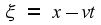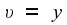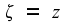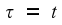As we shall see below, this does not work.

Suppose that a flash of light occurs at the origin of the first frame at zero time, and that the resulting light wavefront expands spherically at the speed of light, which we call c. From the viewpoint of the second frame, traditional theory would tell us that the wavefront also expands spherically as seen in the second frame, and that the sphere's centre moves in the -ξ direction because of the relative motion of the two frames.

This is where Einstein departed from tradition. The motion of a light wavefront is governed by Maxwell's equations, and Maxwell's equations do not depend in any way on the motion of the coordinate system. Einstein supposed that Maxwell's equations would be equally valid in any inertial (that is, non-accelerating) reference frame. This sounds reasonable. The consequence, however, is that the speed of light must be the same in every inertial reference system. That is the point at which we break away from the traditional theory.

If that supposition is true, then the wavefront must be expanding (at the same speed) from the origin of both coordinate frames, since the origins were coincident at the time the light was emitted.

The equation of an expanding spherical surface is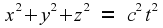Along the x axis, this reduces to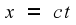So far, so good. But if we map this into the second frame, using the transformation that has just been presented, the equation of the wavefront along the x axis becomes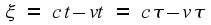so the speed of light in the second frame is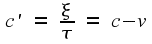This conclusion contradicts the idea that the speed of light should be the same in the two frames. We are therefore forced to conclude that the equations linking the two coordinate systems need modifying.

## Modifying the coordinate transformation

The assumptions thatandare reasonable in most people's minds. There is no motion in those two directions, in our example, so no grounds for assuming that motion would affect those two axes. With motion only in the x direction, we need look only at the equationsWe have already seen that those equations give contradictory conclusions about the speed of light. Thus, we are motivated to look for modified forms of those equations.

It is shown in the Appendix that the transformation that works, in terms of giving the same speed of light in both frames, is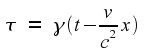where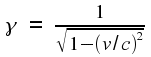(Don't shy away from reading the Appendix. It contains the high school algebra that I mentioned.)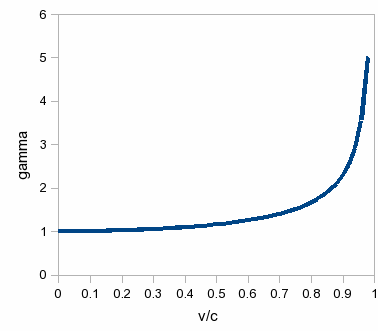Note that the value of γ is always greater than one. (Or equal to one, in the special case of zero relative speed.) For low values of v it is very close to one, so that the transformation between frames is essentially the same as in the non-relativistic case. It is only when v is a substantial fraction of c that the relativistic effects start to become noticeable. This is shown in the graph at right. Even at 50% of the speed of light, γ is only a little bit bigger than 1. As we approach the speed of light, however, γ grows without limit.

## The Inverse Transformation

It is instructive to work out how the transformation goes in the other direction. Starting withlet us solve for x and t. The obvious first step is to write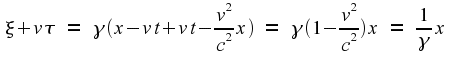The rest of the solution is obvious, so I won't repeat it here. The end result is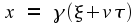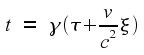This is exactly the same as the original equations, except for the change of sign for v. The change of sign is because, from the point of view of frame 2, frame 1 is moving backwards with speed v.

This is exactly what we should have expected. Indeed, it will be obvious to anyone who has read the Appendix, because the Appendix used these equations to work out the formula for γ. The whole point of relativity is that there is no privileged frame. We can think of frame 2 as moving relative to frame 1, or equally well we can think of frame 1 as moving relative to frame 2. We should get the same result no matter which viewpoint we take.

## Length and time contractions

Think of a rigid rod that is stationary with respect to frame 2, and oriented along the ξ axis. Lengths are, obviously, scaled by a factor γ between the two frames. Thus, this rod appears longer to an observer moving with the rod than it does to an observer in frame 1.

Equivalently, to the observer in frame 1, seeing the rod move past with speed v, the rod appears shorter than its length when at rest. As v approaches the speed of light, the length of the rod appears to shrink down to nothing.

The same scaling factor applies to time intervals. If the observer in the “stationary” frame 1 could observe a clock that is moving with frame 2, the clock would appear to be running fast. Time appears to be going faster in the “moving” frame.

## A numerical example

To get some feel for what is happening here, let us put some numbers on the result. Let us choose the earth for our frame 1. (The earth is not quite an inertial reference frame, because it is following an elliptical orbit, and therefore accelerating, around the sun. For our present purposes, though, it is near enough to being an inertial reference frame.) For frame 2, we choose a spaceship travelling at 80% of the speed of light, relative to the earth. The amount of fuel needed to boost a vehicle to such a speed is beyond our present capabilities, but for the sake of having a good example let us assume that someone, somewhere in the future, has discovered something better than nuclear fission.

We must also assume that people on earth have good enough telescopes, or other measuring instruments, so that each frame can observe the other. (They would have to be pretty good. For such a large relative speed, the spaceship might be out of range before anyone had worked out where to point the telescope.) We also need a method of comparing the clocks in the two frames. This is actually easier: all that is needed is a radio signal that ticks once per second. Radio signals also travel at the speed of light, so there will be a time delay, but it is a delay that can be calculated and corrected for.

For the given relative speed, we can calculateAssume, for the sake of example, that the spaceship is 200 meters long in its direction of travel. To an observer on Earth, it will appear to be only 120 meters long. This is clearly a major difference.

For the time dilation, let us suppose that the spaceship is sending out a “clock” signal by radio that is ticking once per second. On Earth, this will be perceived as a clock that is running 67% fast. The Earth observer will hear 5 ticks every 3 seconds.

Think about someone on the ship who is 20 years old when the ship is passing the Earth. One hundred years later, Earth time, he is still only 80 years old. All of his friends on Earth are dead – they would have been 120 years old if they had survived – but this person has aged by only 60 years. It sounds as if he has discovered some approximation to the fountain of eternal youth.

## Are these contractions real?

When I was in my first university year, a friend of my brother – someone who was still in high school – asked me “Is there a fourth dimension?” I was, I must admit, stumped. The possible answers include “Yes”, “No”, “There are many more than four dimensions”, and “It depends on what you mean by 'dimension'”. The true answer, though, is “You have asked the wrong question.” Once you reach the point of knowing the answer, you also realise that the original question was meaningless.

Relativity is like that. The answer to most questions is “You are asking the wrong question.” Once the question is rephrased, the answer is “mu”.

The previous section seemed to suggest that people on the spaceship live longer, albeit with a weirdly distorted shape, than the people who stayed on Earth, because their biological clocks run slower than their clocks as measured by Earth observers. Remember, though, that from the viewpoint of the people on the ship it is exactly the other way around. The Earth is receding from them at 80% of the speed of light. To them, therefore, the earth has shrunk in one direction, and earth clocks are running slow. The situation is entirely symmetrical.

Meanwhile, the people on the ship have no feeling that their ship has shortened, or that their time scale is distorted. Everything feels normal to them. The contractions do not happen from their point of view. They are only seen by outside observers who are moving at a different speed.

Thus, the contractions that we see in the relativistic equations are an observer effect. They amount to saying “If you are moving relative to the thing you're measuring, your measurements will be distorted”.

That doesn't mean that there aren't practical consequences. People travelling in a high-speed space ship will indeed notice that the universe has been squashed. Not only that; they will get to their destination faster (by their own clocks) than would have been predicted by non-relativistic theories.

# Appendix: Deriving the transformation equations

As in the main text, our starting point is one reference frame (frame 1) with coordinates (x,y,z,t), and a second frame (frame 2), moving with speed v in the +x direction relative to frame 1, with coordinates (ξ,υ,ζ,τ). The coordinate origins are chosen such that the space origins of the two frames are coincident at the time origin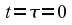.

1. Distances measured at right angles to the relative motion are not affected by the motion. That is, distances in the y and z directions are the same as if there had been no motion.

2. The relationship between the two sets of coordinates is a linear one.

There is, perhaps, no fundamental justification for either of these assumptions, but we have a natural preference for looking for simple solutions before introducing complications. If it turned out that no linear transformation worked, then of course we would have to start looking at nonlinear transformations; but in fact it turns out that we do get a solution with the above assumptions, and furthermore that that solution agrees with what is found by experiment.

With these assumptions, we are looking for a transformation of the form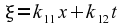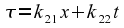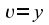where the kij are constants whose values have to be discovered. The last two equations say that we can ignore what is happening in the y and z directions, reducing the problem down to one space dimension and one time dimension.

One thing that should be immediately obvious is that the origin of frame 2, the point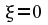, coincides with the moving point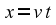in frame 1. That, after all, is simply a restatement of the fact that frame 2 is moving with speed v in the +x direction, relative to frame 1. This means that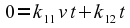from which it follows that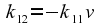. In the main text we will use the symbol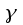to mean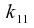, so let us make that change of notation right now. This leaves us with the transformation equations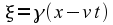We are now down to three constants whose value we have to find.

### A flash of light in the x direction

A typical treatment of this subject considers what will happen when a flash of light occurs at the time that the two origins coincide, and then expands spherically in both frames. The basic assumption is that the wavefront must expand at the same speed, regardless of whether we're measuring it from frame 1 or from frame 2. Although that approach does lead to a solution, it's simpler to reduce the problem down to one spatial dimension, looking only at how the light travels along the x axis.

Consider a case where light is emitted in the +x direction at the time the two origins coincide. In the first frame, the wavefront on the x axis is described by. In the second frame, this maps to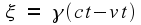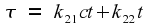Thus the speed of the wavefront, as measured in the second frame, is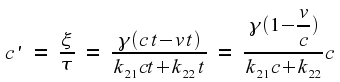To make this exactly equal to c, we need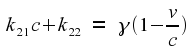Now, let us repeat the experiment, this time with the light being emitted in the -x direction at the time the two origins coincide. In the first frame, the wavefront on the x axis is described by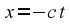. In the second frame, this maps to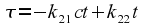Thus the speed of the wavefront, as measured in the second frame, and allowing for the fact that it is moving backwards, is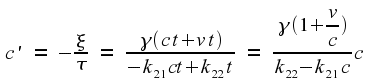To make this exactly equal to c, we need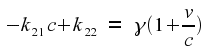For light in the forward direction, we found the constraintAdding these two equations, we get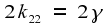and therefore, of course,. This allows us to solve for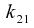. The end result is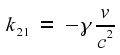This means that the coordinate transformation has the form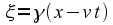and the only remaining thing to be found is the value of γ.

### Shifting our focus to the other reference frame

So far we have taken the viewpoint that frame 2 is moving relative to frame 1. It makes equal sense to treat frame 2 as the “stationary” frame, with frame 1 moving backwards relative to it. (Of course, “stationary” is a label of convenience here. In relativity theories, every inertial frame is an equally good reference frame.) From this viewpoint, the frame 1 coordinates must be able to be expressed in terms of the frame 2 coordinates using the transformation that we have already established. That is,The essential point here is that these are the same equations as were used for the original transformation. The v terms have changed sign, because the relative motion is now in the opposite direction, but otherwise we have the same equations, with the same γ. This is an important point. If all inertial frames are equally good reference points, then the transformation equations should be the same between any pair of inertial frames.

Of course, this will not work for just any arbitrary γ. You would be right in guessing that γ has to be restricted in order to give consistent results. Let us now explore this point.

Expanding out the first equation, we get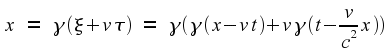which reduces down to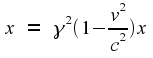Clearly, this can work only if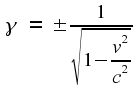Technically, this gives two different solutions for γ. If you look at the transformation equations, though, you will see that the negative solution is no different from the positive solution apart from what is, in effect, a relabelling of the axes to make one of them point in the opposite direction. This is a difference that changes nothing important, so we are justified in deciding to use only the positive solution. Our conclusion, then, is thatNote that this formula has no meaning in real arithmetic if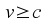. We could play some interesting games with the equations if we decide to allow complex arithmetic, but there are reasons for believing that nothing can be accelerated beyond the speed of light, relative to whichever reference frame you want to use. The reasons for this go beyond the scope of this article, but broadly speaking the reason is that infinite energy would be required. In this article we are looking only at how distance and time measurements are affected by relative motion, but the theory can be extended by looking at how Special Relativity affects the notions of momentum and energy. It turns out that the energy needed to accelerate an object also depends on γ, and γ grows without bound as we approach the speed of light.

There are, it is true, some theories that deal with tachyons, which are hypothetical particles that travel with a speed greater than light speed. It is not yet known whether tachyons exist, but if they do exist they would not contradict the previous paragraph. They don't need to be accelerated beyond the speed of light because their initial speed never was below the speed of light. Indeed, if tachyons exist then it would require infinite energy to decelerate them to the speed of light. The speed-of-light barrier is a barrier in both directions.

### A flash of light expanding spherically

This section is redundant, because we have already worked out the required transformation in the preceding sections. It is included only in case you would prefer to see a different derivation. You can skip it if you wish. I present this alternative derivation with some reluctance, because the mathematics is more complicated in this case; but it seems to be the derivation preferred by textbooks.

Consider the same setup, except that the light has no preferred direction, but expands spherically in all directions. At any instant of time, the wavefront of the light is the surface of a sphere centred on the origin. In frame 1, this sphere is described by the equation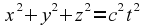In frame 2 the wavefront is also the surface of a sphere centred at the origin, described by the equation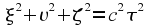which can be rewritten as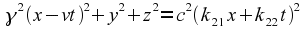We can eliminate y and z by subtracting the frame 1 equation, to give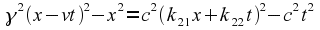Expanding this out, and combining like terms, we get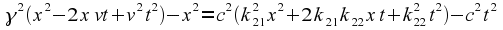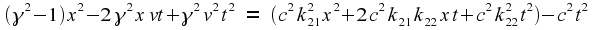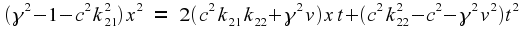What does this mean? If we were talking about light moving only in the x direction, we could substitute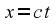to get a result that would turn out not to be interesting. In this case, however, we are looking at a three-dimensional expanding sphere, so that the above should hold for any y and z on the sphere, even though y and z do not appear in the equation. This means, in effect, that we can treat x and t as if they were independent variables.

In that case, though, the only way the equation can hold is if all coefficients are zero. We are therefore forced to conclude that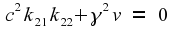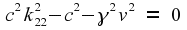The second of these equations can be written asIf we substitute this in the first equation, we get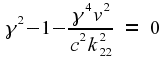orPutting this into the third of our original equations, we get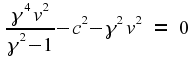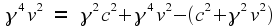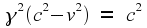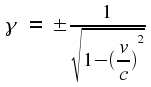(Notice that there are two solutions. We will return to this point later.) Now that we have the solutions for one of the unknowns, the other two follow easily. The results are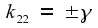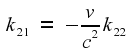Because of the signs, we now have four solutions. Multiple solutions are normal if you start with quadratic equations, but it is possible for some solutions to be spurious, i.e. not true solutions but merely pseudo-solutions introduced because squaring a term eliminated a minus sign. In fact, two of those solutions are spurious. Recall from the previous section that we must haveSubstitutinginto this equation leads to a contradiction, so we are left with only the case whereandhave the same sign. That cuts the possibilities down to only two solutions: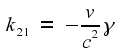Of these, only the case where is positive is interesting. The other case amounts to a simple relabelling of the frame 2 axes, i.e. it is not really a separate solution.

Finally, then, the coordinate transformation iswhere